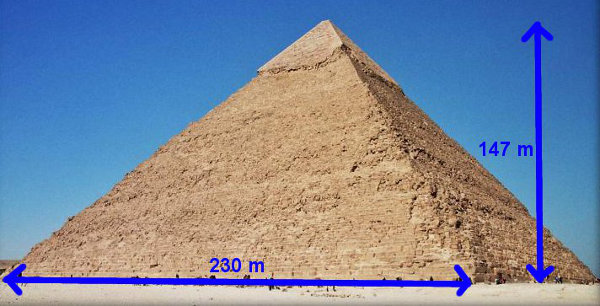# Volume Of Pyramids

Pyramids are 3-dimensional solids where the cross-section reduces in size to a point.

Examples are square-based pyramids (e.g. Egyptian pyramids), triangle-based pyramids (tetrahedrons) and circle-based pyramids (cones).

Because the pyramid is one-third of the volume of an equal-dimensioned prism, the general rule for volume of a pyramid is:

Volume of Pyramid = 13 × Area of Cross-section × Height

## Example One - Volume of Stone in an Egyptian PyramidThe Great Pyramid at Giza in Egypt has a square base with a side of 230 metres and a vertical height of 147 metres. What is the volume of stone?

The cross-section is a square of decreasing size.

Volume of Pyramid = 13 × Area of Cross-section × Height
= 13 × Area of Square × Height
= 13 × (S × S) × H
= 13 × (230 × 230) × 147
= 2 592 100 m3 of stone

## Did You Know That...?

In your retina (the curved back part of your eye) are hundreds of tiny cone cells for colour vision. They are mainly concentrated in the middle of the retina.

(Try looking at something colourful by first looking directly at it, and then looking side on. It should be brighter if you look directly at it.)

Cone cells also require a higher intensity of light to function than do the other retinal cells called rod cells for black-and-white vision which are mainly around the periphery of the retina.

(When you are lying in bed tonight with the lights out, look at colourful objects in your room. Do they appear more greyish and lacking in colour?)

## Example Two - Volume of CrayonA crayon has 2 parts - a cylinder and a cone-shaped top. The cylinder has a diameter of 10 mm and a length of 70 mm. The cone has a vertical height of 8 mm. What is the volume of one crayon? (Use π = 3.14.)

Volume of Prism (Cylinder)
The prism cross-section is a circle of the same size.
Volume of Prism = Area of Cross-section × Height
= Area of Circle × Height
= π r2 × H
= 3.14 × 5 × 5 × 10
= 785 mm3

Volume of Pyramid (Cone)
The pyramid cross-section is a circle of decreasing size.
Volume of Pyramid = 13 × Area of Cross-section × Height
= 13 × Area of Circle × Height
= 13 × π r2 × H
= 13 × 3.14 × 5 × 5 × 8
= 209.3 mm3

## Question - I Scream for Ice-cream

How much ice-cream is in a cone with a diameter of 6 cm and a vertical height of 12 cm?